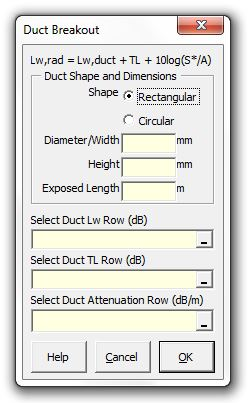### Strutt Help

Duct Breakout    1/1, 1/3

Strutt|Mechanical Services|Duct Breakout|Duct Breakout (Allen) inserts the sound power level of sound transmitted through a duct wall into the active row of the worksheet.The sound power level associated with sound transmitted through the duct walls and then radiated from the exterior surface of the duct wall is given by:

L_(W,radiated) = L_(W,duct) + TL + 10log_10((S"*")/A)

where,
L_(W,radiated) is the sound power level of sound radiated from the outside surface of duct walls, dB
L_(W,duct) is the sound power level of sound inside duct, dB
TL is the duct transmission loss, dB (this can be input manually or via Duct TL function). Note: TL values must be negative for formula to calculate correctly. The formula will not work correctly for low TLs and is designed to calculate sheet metal duct breakout.
S"*" = PL"*" is the effective surface area of outside sound-radiating surface of duct, m2
P is the duct perimeter (m)
L*=(gamma^L-1)/ln(gamma) is the effective duct length (m)
gamma=10^(-alpha//10) is a coefficient related to the duct attenuation rate alpha (dB/m)
A is the cross-sectional area of inside of duct, m2

References:

• ASHRAE Handbook (2007), p47.21